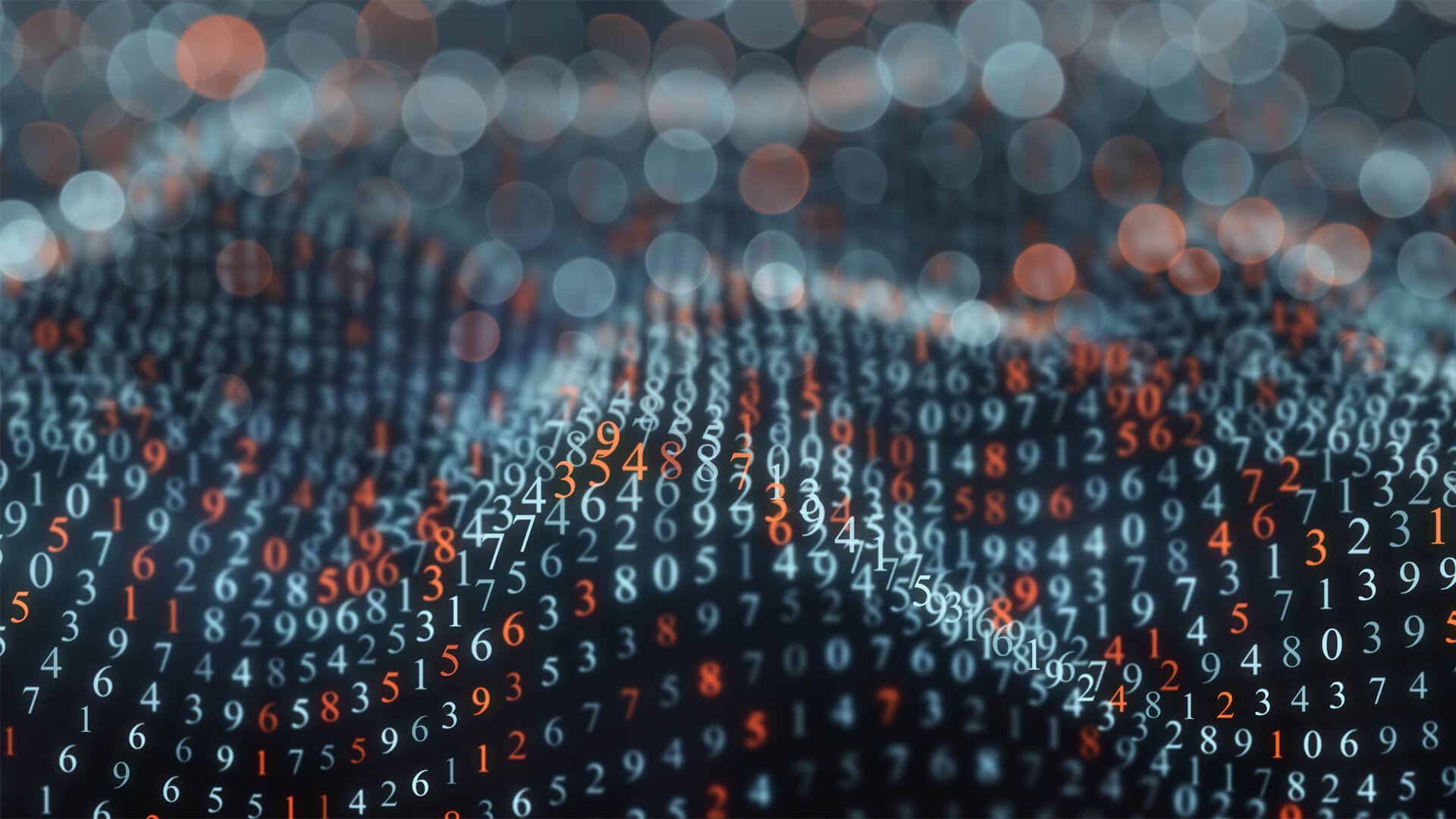## Stochastic Process by Mr. V.Rama Krishna Sharma M.Tech, (Ph. D) Stochastic Process gives the basic understanding of random process and its characteristics along with the response of linear time invariant systems. Further, its study is essential for communication and signal processing areas. Code : SPJHECE21 Recommended For : JNTU B. Tech ECE 2nd Year 1st Semester

#### After Learning this subject,you should be able to

• Basic understanding of random process and its characteristics along with the response of linear time invariant systems. Further, its study is essential for communication and signal processing areas.

## Curriculum 8:44:49   units   |   7

• 0:19:57

UNIT 4
Stochastic processes Introduction: Signals & Stochastic processes Introduction- part 1, Signals & Stochastic processes Introduction- part 2

• 1:59:38

UNIT 4.x
PRE-REQUISITES: PRE-REQUISITES FOR STOCHASTIC (RANDOM) PROCESS, What is a Random Variable, What is a Random Variable part 1, Probability distribution Function and Properties of Distribution Function, Expression for distribution function, Plot of distribution Function, Probability Density Function, Numerical Example for density function, PRE-REQUISITES FOR STOCHASTIC (RANDOM) PROCESS, OPERATIONS ON, Moments, Moments about the Mean Value Part 1, Moments about the Mean Value Part 2, Moments about the Mean Value Part 3, Moments about the Mean Value Part 4

• 1:26:24

UNIT 4.1
Random Processes-Temporal Characteristics: Random processes temporal characteristics, Random Process Concept, Classification of Processes, CRP & DRP & CRS & DRS, Deterministic and Nondeterministic Processes, Distribution and Density Functions, Concept of Stationarity First- Order Stationarity, Second- Order and Wide-Sense Stationarity, (N-Order) and Strict-Sense Stationarity, Concept of Statistical Independence, Time Averages, Ergodicity, Mean-Ergodic, Correlation Ergodic

• 1:14:40

UNIT 4.2
Autocorrelation Function: Autocorrelation Function (ACF), Properties Of ACF- Part 1, Properties Of ACF- Part 2, Cross Correlation Function (CFF), Properties Of CCF, Covariance Functions- part 1, Covariance Functions- part 2, Gaussian Random Process- part 1, Gaussian Random Process- part 2

• 1:26:21

UNIT 4.3
Poisson Random Process: Poisson Random Process- part 1, Poisson Random Process- part 2, Random Signal- Part 1, Random Signal- Part 2, Mean Value of System Response, Mean-squared Value of System Response, Autocorrelation Function of Response, Cross-Correlation Of Functions Of Input And Output, Problem 1, Problem 2

• 1:16:18

UNIT 5.1
Random Processes-Spectral Characteristics: Random Processes, Power Spectrum & its Properties - part 1, Power Spectrum & its Properties - part 2, Properties of PDS- part 1, Properties of PDS- part 2, Properties of PDS- part 3, Properties of PDS- part 4, The Cross-Power Spectrum Density (CPSD)

• 1:01:31

UNIT 5.2
Properties of CPSD: Properties of CPSD, Relationship between CPSD & CCF- part 1, Relationship between CPSD & CCF- part 2, Spectral Characteristics of System Response, Power Density Spectrum of Response, Cross-Power Density Spectrums of Input and Output, Autocorrelation function, Cross-Power Spectrum Density